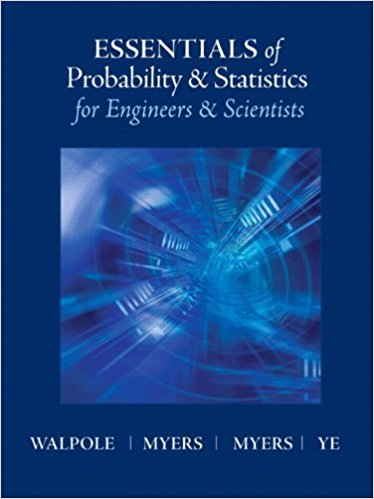×
×

# Solutions for Chapter 2: Random Variables, Distributions and Expectations## Full solutions for Essentials of Probability & Statistics for Engineers & Scientists | 1st Edition

ISBN: 9780321783738Solutions for Chapter 2: Random Variables, Distributions and Expectations

Solutions for Chapter 2
4 5 0 298 Reviews
29
2
##### ISBN: 9780321783738

Essentials of Probability & Statistics for Engineers & Scientists was written by and is associated to the ISBN: 9780321783738. Since 135 problems in chapter 2: Random Variables, Distributions and Expectations have been answered, more than 15144 students have viewed full step-by-step solutions from this chapter. This expansive textbook survival guide covers the following chapters and their solutions. This textbook survival guide was created for the textbook: Essentials of Probability & Statistics for Engineers & Scientists, edition: 1. Chapter 2: Random Variables, Distributions and Expectations includes 135 full step-by-step solutions.

Key Statistics Terms and definitions covered in this textbook
• 2 k factorial experiment.

A full factorial experiment with k factors and all factors tested at only two levels (settings) each.

• Analytic study

A study in which a sample from a population is used to make inference to a future population. Stability needs to be assumed. See Enumerative study

• Axioms of probability

A set of rules that probabilities deined on a sample space must follow. See Probability

• Bimodal distribution.

A distribution with two modes

• Bivariate normal distribution

The joint distribution of two normal random variables

• C chart

An attribute control chart that plots the total number of defects per unit in a subgroup. Similar to a defects-per-unit or U chart.

• Combination.

A subset selected without replacement from a set used to determine the number of outcomes in events and sample spaces.

• Conditional variance.

The variance of the conditional probability distribution of a random variable.

• Continuity correction.

A correction factor used to improve the approximation to binomial probabilities from a normal distribution.

• Continuous uniform random variable

A continuous random variable with range of a inite interval and a constant probability density function.

• Correlation matrix

A square matrix that contains the correlations among a set of random variables, say, XX X 1 2 k , ,…, . The main diagonal elements of the matrix are unity and the off-diagonal elements rij are the correlations between Xi and Xj .

• Critical value(s)

The value of a statistic corresponding to a stated signiicance level as determined from the sampling distribution. For example, if PZ z PZ ( )( .) . ? =? = 0 025 . 1 96 0 025, then z0 025 . = 1 9. 6 is the critical value of z at the 0.025 level of signiicance. Crossed factors. Another name for factors that are arranged in a factorial experiment.

• Defects-per-unit control chart

See U chart

• Design matrix

A matrix that provides the tests that are to be conducted in an experiment.

• Designed experiment

An experiment in which the tests are planned in advance and the plans usually incorporate statistical models. See Experiment

• Discrete random variable

A random variable with a inite (or countably ininite) range.

• Distribution function

Another name for a cumulative distribution function.

• F distribution.

The distribution of the random variable deined as the ratio of two independent chi-square random variables, each divided by its number of degrees of freedom.

• Fraction defective control chart

See P chart

• Goodness of fit

In general, the agreement of a set of observed values and a set of theoretical values that depend on some hypothesis. The term is often used in itting a theoretical distribution to a set of observations.

×